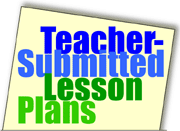Home >> A Tsl >> Archives >> 03 1 >> Talking About Your Name in Math Terms

## Search formTalking About Your Name in Math Terms

Subjects

• Geometry

• K-2
• 3-5
• 6-8
• 9-12

Brief Description

This lesson is designed to promote the use and understanding of the language associated with mathematics.

Objectives

• examine their names for mathematical concepts found therein.
• express their names in mathematical terms/language.
• look for math in other words and phrases.

Keywords

math, vocabulary, imagination, creative

• paper
• pencils
• dictionaries
• imagination!

The Lesson

This is a fun lesson to use at the beginning of the year, especially for math teachers; but it can be used at any time of year, and can be repeated during the year as students build analytical thinking skills and their math vocabulary.

Begin the lesson by writing your own name (or perhaps the school's name) in LARGE BLOCK LETTERS on the board or a chart. Stand back and study the name, then make grade-appropriate mathematical comments about it; for example, I might begin by saying "My name is PAULINA. I can make a lot of mathematical comments about my name. There are seven letters in PAULINA. The L is the middle letter. The L is a right angle. There are two A's; the letter A is a congruent letter. The P and the A's have a similar property; they all contain one enclosed space. The I is vertical, but if it was rotated it would be horizontal..." The idea is to include as many mathematical descriptions as you can think of for your name.

Have students write a word -- such as their own name -- in block letters. Alternatively, you might let students work in pairs or give individual students a word such as the name of their town or unit of study (such as ENVIRONMENT or GEOMETRY). Give students time to analyze the word from a mathematical perspective according to their level. Young students will recognize numbers and shapes and be able to talk about them; older students will be able to locate and write/discuss vertices, angles of depression, converging lines, corresponding angles, radiant points, and so on.

Math vocabulary will depend upon the grade/math level of the students. Younger students will use terms such as same as, sloped, square, and lines going the same way while older students might use other words meaning the same things such as congruent, diagonal, quadrilateral, and parallel.

Extending the lesson.

• In subsequent lessons, a small prize might be given for the student who finds the most mathematical comments about a given word.
• Parents can help if the lesson is sent home for homework.

Assessment

The quality and the quantity of the comments -- either spoken or written -- will be assessed; the students' skills in this area should increase with successive lessons.

Submitted By

Paulina Jurkijevic, Bakewell Primary School in Palmerston, Northern Territory (Australia)

Education WorldÂ®